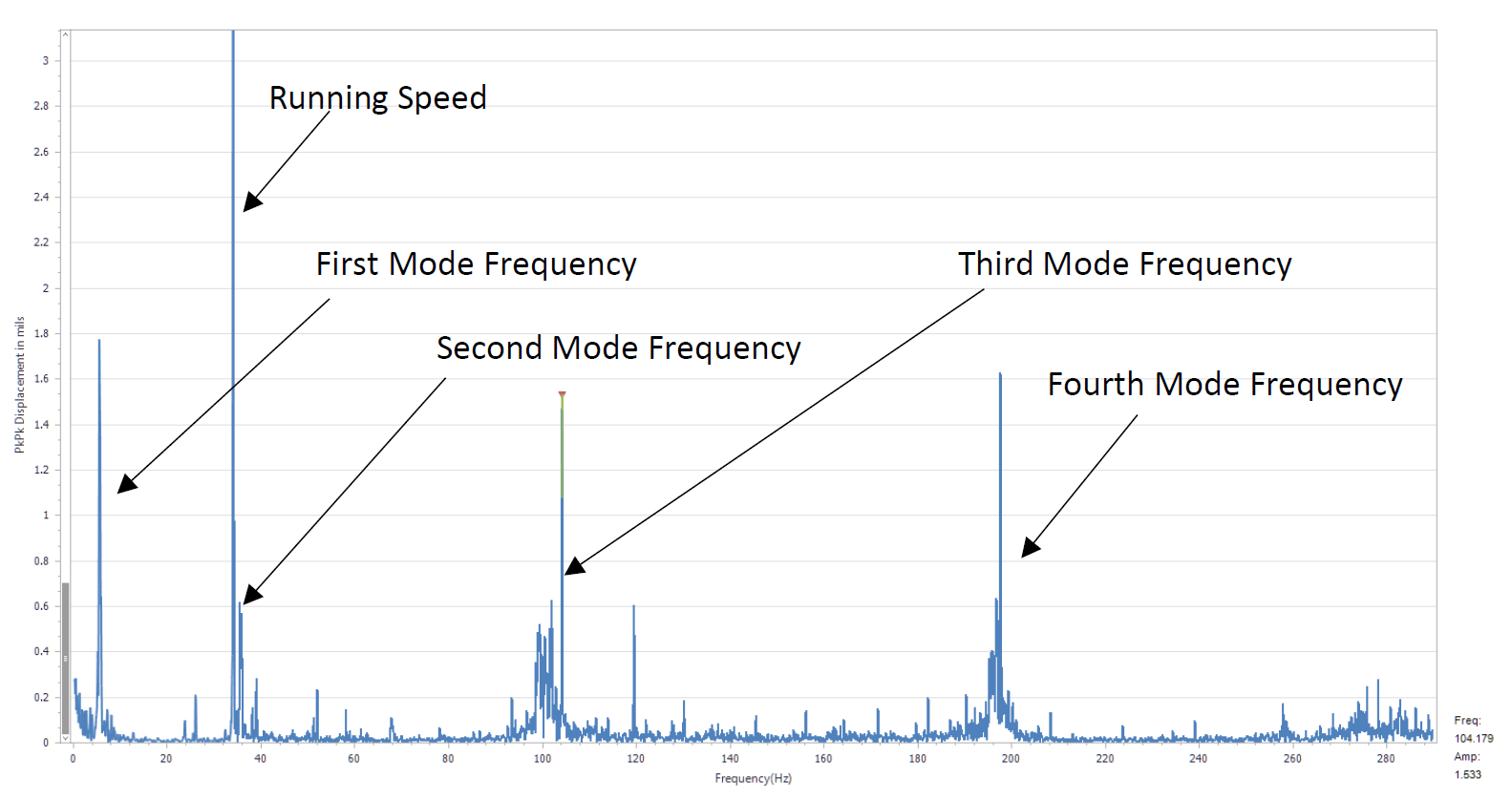Select Page

RDI CASE STUDY

### Measurement of a Cantilever Beam on a Machinery Vibration Trainer

The Iris M™ was used to collect data on a Machine Vibration Analysis Multi-Mode Trainer, Model MVAT6 by V-TEK Associates. The model was equipped with a Resonance Study beam and is driven by a ¼ HP PWM variable speed DC drive with a max speed of 3,400 RPM.1 The purpose was to analyze the mode shapes of the resonance study beam. This was done by measuring the displacement of each mode shape frequency at incremental distances from the clamped end of the beam. RDI’s Motion Amplification™ software was used to show the mode shapes at particular frequencies while undergoing vibration.2 The trainer was operated at a speed of 2,082 RPM or 37.4 Hz. The beam length is 19.13 in (0.486m) and is marked at 1-inch increments starting from the supported end.Figure 1

Theory

The movement of the vibrating cantilever beam can be determined from the Bernoulli-Euler Equation3Setting the appropriate boundary conditions for a fixed-free beam, the natural frequencies can be determined to be as follows3:The given beam is made of aluminum and has the following measured properties.This gives the following theoretical natural frequencies of vibration for the cantilever beam based on measured properties.These frequencies can now be compared to those measured from the resonance beam.

Measurements

Measurements of the vertical motion of the beam were made at 1-inch increments along the length of the beam. Figure 2 shows the resonance study beam with the 1-inch spacing. Figure 3 shows the Motion Amplification™ Analysis software with the boxes indicating the points of each measurement. A key point to using the Iris M system, especially in this scenario, is the ability to measure without attaching a contact sensor to the target. In this case, it is key that mass is not added to the system since the cantilever beam has such a small mass. Adding a contact sensor where the sensor is appreciable compared to the mass of the beam would fundamentally alter the dynamic properties of the beam as well as make it asymmetric. In this case, the Iris M is an ideal measurement tool. While the beam is modeled as a cantilever there is some expected deviation from a theoretical true cantilever setup, for example, the support structure having some vibration on the fixed end resulting in a less stiff structure, lowering frequencies.

The motor was run at 2082 RPM or 37.4 Hz, slightly off the 2nd natural frequency, to excite the natural frequencies but not drive the beam at one particular resonance. All measurements were made while driving the motor at 2082 RPM.Figure 2Figure 3

The frequencies were identified in the spectrum as shown in Figure 4. These frequencies were also confirmed by analyzing the videos filtered at the respective frequencies and shown to behave in a manner consistent with the identified mode shape.2Figure 4

The measured frequencies are:This gives an error from the calculated values of 8.0%, 9.7%,  5.3% and 8.4% respectively. A source of error could result from the beam not be 100% fixed as the support structure for the beam vibrates as well.

The amplitudes for each of the frequencies were measured at each point along the bar. Those values are summarized in Table 1.Table 1

The amplitudes can then be plotted to show the shapes of the bending modes. The measured values are shown plotted in Figure 5. They closely resemble the classical examples of modes shapes expected for a cantilever beam fixed on one end and free on another. Figure 6 shows an example of the first four bending modes from theoretical values.4 This is provided as a reference for comparison to the measured values showing the measured values to be in good agreement with the theoretical values.Figure 5Figure 6

1. V-TEK ASSOCIATES MACHINE TRAINER. Available at: https://vtekassociates.com/Trainers.html. (Accessed: 17th July 2017)
2. RDI Technologies. A Study of a Machinery Trainer.
3. Structural Optimization of Cantilever Beam in Conjunction with Dynamic Analysis. ResearchGate Available at: https://www.researchgate.net/publication/273452232_Structural_Optimization_of_Cantilever_Beam_in_Conjunction_with_Dynamic_Analysis.
4. Euler–Bernoulli beam theory. Wikipedia (2017).